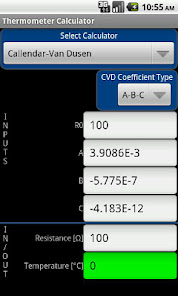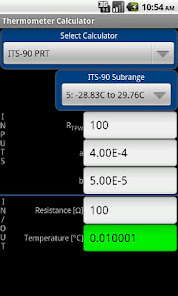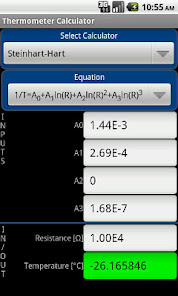# Thermometer Calculator

5K+EveryoneCalculate temperature from resistance or resistance from temperature given calibration values for:
★ PRT using Callendar-Van Dusen equation
★ PRT using ITS-90
★ Thermistor using Steinhart-Hart equation

Save and load thermometer coefficients. Convert CVD coefficients between a-b-c and α-δ-β equations.

Designed for measurement scientists and engineers.

a Pocket Metrologist(TM) tool
Updated on
Apr 18, 2021

## Data safety